Professor Murray

Sequences

Slide Duration:

Integration by Parts

24m 52s

Intro
0:00
Important Equation
0:07
Where It Comes From (Product Rule)
0:20
Why Use It?
0:35
Lecture Example 1
1:24
Lecture Example 2
3:30
Shortcut: Tabular Integration
7:34
Example
7:52
Lecture Example 3
10:00
Mnemonic: LIATE
14:44
Ln, Inverse, Algebra, Trigonometry, e
15:38
-1
-2
Integration of Trigonometric Functions

25m 30s

Intro
0:00
Important Equation
0:07
Powers (Odd and Even)
0:19
What To Do
1:03
Lecture Example 1
1:37
Lecture Example 2
3:12
Half-Angle Formulas
6:16
Both Powers Even
6:31
Lecture Example 3
7:06
Lecture Example 4
10:59
-1
-2
Trigonometric Substitutions

30m 9s

Intro
0:00
Important Equations
0:06
How They Work
0:35
Example
1:45
Remember: du and dx
2:50
Lecture Example 1
3:43
Lecture Example 2
10:01
Lecture Example 3
12:04
-1
-2
Partial Fractions

41m 22s

Intro
0:00
Overview
0:07
Why Use It?
0:18
Lecture Example 1
1:21
Lecture Example 2
6:52
Lecture Example 3
13:28
-1
-2
Integration Tables

20m

Intro
0:00
Using Tables
0:09
Match Exactly
0:32
Lecture Example 1
1:16
Lecture Example 2
5:28
Lecture Example 3
8:51
-1
-2
Trapezoidal Rule, Midpoint Rule, Left/Right Endpoint Rule

22m 36s

Intro
0:00
Trapezoidal Rule
0:13
Graphical Representation
0:20
How They Work
1:08
Formula
1:47
Why a Trapezoid?
2:53
Lecture Example 1
5:10
Midpoint Rule
8:23
Why Midpoints?
8:56
Formula
9:37
Lecture Example 2
11:22
Left/Right Endpoint Rule
13:54
Left Endpoint
14:08
Right Endpoint
14:39
Lecture Example 3
15:32
-1
-2
Simpson's Rule

21m 8s

Intro
0:00
Important Equation
0:03
Estimating Area
0:28
Difference from Previous Methods
0:50
General Principle
1:09
Lecture Example 1
3:49
Lecture Example 2
6:32
Lecture Example 3
9:07
-1
-2
Improper Integration

44m 18s

Intro
0:00
Horizontal and Vertical Asymptotes
0:04
Example: Horizontal
0:16
Formal Notation
0:37
Example: Vertical
1:58
Formal Notation
2:29
Lecture Example 1
5:01
Lecture Example 2
7:41
Lecture Example 3
11:32
Lecture Example 4
15:49
Formulas to Remember
18:26
Improper Integrals
18:36
Lecture Example 5
21:34
Lecture Example 6 (Hidden Discontinuities)
26:51
-1
-2
Section 2: Applications of Integrals, part 2
Arclength

23m 20s

Intro
0:00
Important Equation
0:04
Why It Works
0:49
Common Mistake
1:21
Lecture Example 1
2:14
Lecture Example 2
6:26
Lecture Example 3
10:49
-1
-2
Surface Area of Revolution

28m 53s

Intro
0:00
Important Equation
0:05
Surface Area
0:38
Relation to Arclength
1:11
Lecture Example 1
1:46
Lecture Example 2
4:29
Lecture Example 3
9:34
-1
-2
Hydrostatic Pressure

24m 37s

Intro
0:00
Important Equation
0:09
Main Idea
0:12
Different Forces
0:45
Weight Density Constant
1:10
Variables (Depth and Width)
2:21
Lecture Example 1
3:28
-1
-2
Center of Mass

25m 39s

Intro
0:00
Important Equation
0:07
Main Idea
0:25
Centroid
1:00
Area
1:28
Lecture Example 1
1:44
Lecture Example 2
6:13
Lecture Example 3
10:04
-1
-2
Section 3: Parametric Functions
Parametric Curves

22m 26s

Intro
0:00
Important Equations
0:05
Slope of Tangent Line
0:30
Arc length
1:03
Lecture Example 1
1:40
Lecture Example 2
4:23
Lecture Example 3
8:38
-1
-2
Polar Coordinates

30m 59s

Intro
0:00
Important Equations
0:05
Polar Coordinates in Calculus
0:42
Area
0:58
Arc length
1:41
Lecture Example 1
2:14
Lecture Example 2
4:12
Lecture Example 3
10:06
-1
-2
Section 4: Sequences and Series
Sequences

31m 13s

Intro
0:00
Definition and Theorem
0:05
Monotonically Increasing
0:25
Monotonically Decreasing
0:40
Monotonic
0:48
Bounded
1:00
Theorem
1:11
Lecture Example 1
1:31
Lecture Example 2
11:06
Lecture Example 3
14:03
-1
-2
Series

31m 46s

Intro
0:00
Important Definitions
0:05
Sigma Notation
0:13
Sequence of Partial Sums
0:30
Converging to a Limit
1:49
Diverging to Infinite
2:20
Geometric Series
2:40
Common Ratio
2:47
Sum of a Geometric Series
3:09
Test for Divergence
5:11
Not for Convergence
6:06
Lecture Example 1
8:32
Lecture Example 2
10:25
Lecture Example 3
16:26
-1
-2
Integral Test

23m 26s

Intro
0:00
Important Theorem and Definition
0:05
Three Conditions
0:25
Converging and Diverging
0:51
P-Series
1:11
Lecture Example 1
2:19
Lecture Example 2
5:08
Lecture Example 3
6:38
-1
-2
Comparison Test

22m 44s

Intro
0:00
Important Tests
0:01
Comparison Test
0:22
Limit Comparison Test
1:05
Lecture Example 1
1:44
Lecture Example 2
3:52
Lecture Example 3
6:01
Lecture Example 4
10:04
-1
-2
Alternating Series

25m 26s

Intro
0:00
Main Theorems
0:05
Alternation Series Test (Leibniz)
0:11
How It Works
0:26
Two Conditions
0:46
Never Use for Divergence
1:12
Estimates of Sums
1:50
Lecture Example 1
3:19
Lecture Example 2
4:46
Lecture Example 3
6:28
-1
-2
Ratio Test and Root Test

33m 27s

Intro
0:00
Theorems and Definitions
0:06
Two Common Questions
0:17
Absolutely Convergent
0:45
Conditionally Convergent
1:18
Divergent
1:51
Missing Case
2:02
Ratio Test
3:07
Root Test
4:45
Lecture Example 1
5:46
Lecture Example 2
9:23
Lecture Example 3
13:13
-1
-2
Power Series

38m 36s

Intro
0:00
Main Definitions and Pattern
0:07
What Is The Point
0:22
0:45
Interval of Convergence
2:42
Lecture Example 1
3:24
Lecture Example 2
10:55
Lecture Example 3
14:44
-1
-2
Section 5: Taylor and Maclaurin Series
Taylor Series and Maclaurin Series

30m 18s

Intro
0:00
Taylor and Maclaurin Series
0:08
Taylor Series
0:12
Maclaurin Series
0:59
Taylor Polynomial
1:20
Lecture Example 1
2:35
Lecture Example 2
6:51
Lecture Example 3
11:38
Lecture Example 4
17:29
-1
-2
Taylor Polynomial Applications

50m 50s

Intro
0:00
Main Formulas
0:06
Alternating Series Error Bound
0:28
Taylor's Remainder Theorem
1:18
Lecture Example 1
3:09
Lecture Example 2
9:08
Lecture Example 3
17:35
-1
-2
Bookmark & Share Embed

## Copy & Paste this embed code into your website’s HTML

Please ensure that your website editor is in text mode when you paste the code.
(In Wordpress, the mode button is on the top right corner.)
×
• - Allow users to view the embedded video in full-size.
Since this lesson is not free, only the preview will appear on your website.

• ## Related Books1 answer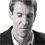Last reply by: Dr. William MurrayWed Dec 28, 2016 11:13 AMPost by Acme Wang on December 27, 2016Hi Professor,Just a bit confused about Example II. The question does not say take the limit as n goes to infinity, how could you just assume that? :):) Really nice video! Thank you very much!1 answerLast reply by: Dr. William MurrayWed Aug 19, 2015 10:06 AMPost by Mitchell Mayberry on August 17, 2015Are most of the problems in the sequences section for an average Calc 2 class going to be taking the limit?1 answerLast reply by: Dr. William MurrayWed Jul 1, 2015 8:50 AMPost by Rafael Mojica on June 26, 2015I dont understand why we went from sequences to taking the limit.1 answerLast reply by: Dr. William MurrayFri Jun 27, 2014 5:02 PMPost by robert moreno on June 27, 2014in ex.4 can you use l'hospital's rule after you use the conjugate?1 answerLast reply by: Dr. William MurraySat Apr 19, 2014 5:37 PMPost by Taylor Wright on April 16, 2014In Ex1, how could (an-2)(an+1) be less than or equal to zero if an-2<0 and an+1>0  ?   When multiplied together couldn't they only produce a negative value, therefore (an-2)(an+1) should only be < 0   ?   I don't see how their product could ever equal zero.  Thank you.1 answerLast reply by: Dr. William MurrayMon Oct 21, 2013 7:45 PMPost by Nommel Meless Ghislain Djedjero on October 17, 2013I meant not by 3?1 answerLast reply by: Dr. William MurrayMon Oct 21, 2013 7:45 PMPost by Nommel Meless Ghislain Djedjero on October 17, 2013How do you know that the sequence is bounded above by 2 and not by 1 ?1 answerLast reply by: Dr. William MurrayFri Aug 31, 2012 5:39 PMPost by Brian Raaflaub on July 27, 2012How do you determine the divergence or convergence of the sequence sin(2n)/(1+(n^1/2)) ??1 answerLast reply by: Dr. William MurrayMon May 13, 2013 10:58 AMPost by Real Schiran on November 4, 2011Cool !!1 answerLast reply by: Dr. William MurrayMon May 13, 2013 10:57 AMPost by khadar mire on October 25, 2011very helpful.thanks eductors team1 answerLast reply by: Dr. William MurrayMon May 13, 2013 10:54 AMPost by nizar ayadi on May 1, 2011How can We determine if the sequense n^2 e ^ (-n) is convergent or divergent ?1 answerLast reply by: Dr. William MurrayMon May 13, 2013 10:50 AMPost by mary setlock on July 5, 2010:) i just love BC calculus. what a great way to spend a monday night!happy integrating!!!!!!1 answerLast reply by: Dr. William MurrayMon May 13, 2013 10:48 AMPost by Collin Wilson on March 21, 2010Hello, in example 2, the notation should (for completeness) should be lim (n-> inf) 3n+5n^2 / 2n^2 +6 :)

### Sequences

Main definitions and theorem:

Let {an} be a sequence.

Definitions:

• {an} is monotonically increasing means an+1an for all n.

• {an} is monotonically decreasing means an+1an for all n.

• {an} is monotonic means {an} is one or the other.

• {an} is bounded means there exists M such that |an| ≤ M for all n.

Theorem: If {an} is bounded and monotonic, then it converges.

Hints and tips:

• In determining whether a sequence converges or diverges and what it converges or diverges to, you can ignore the first few terms. What is important is what the later terms do.

• Don’t try to analyze sequences by plugging numbers into a calculator. This is extremely unreliable.

• You often use the “bounded monotonic” theorem to analyze sequences that are defined recursively, that is, an+1 is defined in terms of an.

• When analyzing fractions, focus on the biggest term in the top and the biggest term in the bottom. Remember the following ranking of functions: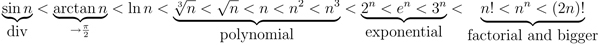• You can use L’Hôpital’s Rule for situations of the form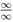or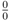. If you have 0 · ∞, you must put it into fraction form before you can use L’Hôpital. The same goes for 1 : Write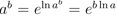. Then you can often sort out the exponent using L’Hôpital.

• When you have an expression of the form a − b, especially when square roots are involved, it is often useful to multiply by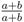to take advantage of the identity (a − b)(a + b) = a² − b² .

### Sequences

Lecture Slides are screen-captured images of important points in the lecture. Students can download and print out these lecture slide images to do practice problems as well as take notes while watching the lecture.

• Intro 0:00
• Definition and Theorem 0:05
• Monotonically Increasing
• Monotonically Decreasing
• Monotonic
• Bounded
• Theorem
• Lecture Example 1 1:31
• Lecture Example 2 11:06
• Lecture Example 3 14:03

### Transcription: Sequences

We are here to try some more examples of finding limits of sequences.0000

The first one is the sqrt(n2 + 6n) - n.0006

There is a trick here when you see square roots and you see them being added and subtracted with things.0014

The trick is to multiply the top and bottom by the conjugate.0023

What I mean by the conjugate is if you have an expression like a + b,0040

You multiply it by a - b/a - b.0046

So, if you have a + b, you multiply it by a - b,0052

If you have a - b, you multiply it by a + b.0058

The point of doing that is to take advantage of this difference of squares formula.0062

Remember a + b × a - b = a2 - b2.0067

What that does is it squares out the expressions for you and that can often simply a square root formula.0075

Let us try that out with this example.0083

We have sqrt(n2 + 6n) - n.0088

I want to multiply that by its conjugate, the sqrt(n2+6n)+n.0094

To pay for that multiplication, I have to do some division by the same expression,0104

sqrt(n2+6n)+n.0110

The point of that is that in the numerator we get this a2-b2 expression.0115

We get the sqrt(n2+6n)2, so that is just n2+6n.0124

Minus n2.0134

Then that same denominator.0140

sqrt(n2+6n) + n.0142

This simplifies nicely down to 6n/sqrt(n2+6n) + n.0150

We are not done yet with this because we still have something that is not so obvious.0160

We have 6n in the numerator, some square root stuff in the denominator.0167

The key thing to remember is to go through and look at the top and bottom and see what the biggest thing is in both the numerator and the denominator.0171

In the top I see the biggest thing here is n.0180

In the bottom, well I see sqrt(n2), that is approximately n.0186

You know there is a 6n next to it.0190

The n2 is bigger than the 6n, so the sqrt(n2) is appromixately n.0194

Then of course we have an n in the denominator.0200

The biggest thing top and bottom would be n.0204

What I want to do is divide top and bottom by n.0206

That will give me just 6 in the numerator and then in the denominator,0213

sqrt(n2+6n)/n + 1.0220

Sqrt(n2+6n), I can write that as,0230

Well I can make that a big square root.0236

n2+6n.0241

Now if I want to make this n part of the square root, I have to change it to n2.0244

That in turn becomes 1+6/n.0248

This whole thing becomes 6/sqrt(1+6/n)+1.0258

Now it is in a format that is very conducive to letting n go to infinity.0269

As n goes to infinity, the 6/n goes to 0.0275

We get 6/sqrt(1) which is just 1 + 1.0278

So, 6/2 and we get our answer, that the sequence converges to 3.0283

There are a couple clever idea in that one.0291

The first was that we had a square root of something minus something.0296

The trick to handling that is by multiplying that by its conjugate.0301

We multiplied it by the same expression with a plus there.0306

To pay for that multiplication, we had to do a division.0311

The point of multiplying by the conjugate,0315

Is we can exploit this difference of squares formula.0319

We get the sqrt(n2+6n)2, that gets rid of the square root there.0324

We get n2, and we get this difference of squares formula,0333

Which simplifies down into 6n.0339

That was the first trick, multiplying by the conjugate.0342

The second trick was looking at the expression we got, and then dividing top and bottom by the biggest term that we saw.0346

Then recognizing that when we had the square root of n2, that was really approximately n,0350

The biggest term in top and bottom was n.0360

We divide top and bottom by n.0362

Then do some algebra to simplify it, and we finally get our answer.0366

Our final example here is to calculate the limit of 1 + 1/n2n.0000

This is an example that is quite common in Calculus homework sets.0007

It is also one that students often make mistakes on.0013

People think that as n goes to infinity, 1+1/n ought to go to 1 + 0.0016

So, you are 1+0infinity, so that is 1infinity,0026

And people think that goes to 1.0035

That is a very common response that students give to problems of this form.0038

That is flat wrong.0043

Let me emphatically warn you away from making that mistake.0046

This is actually something a lot more subtle.0053

If you see an expression that looks like 1 to the infinity,0056

There is something deeper going on there.0060

We are actually going to need some more sophisticated tools for that.0063

The trick here is when you have an expression of the form ab,0070

Often, a very useful way to write that is,0078

Write it in the form, eln(ab).0087

The point of that is that the ln(ab),0097

Is the same as bln(a).0100

Natural log is a way of separating an exponent out to the side of an expression,0104

So that it is no longer an exponent.0113

Then we have to put an e in there to cancel off the natural log.0117

The e is kind of paying for the natural log,0120

While the natural log is doing the actual work of moving the exponent out of the exponent,0123

So that we just have a multiplication.0127

Then, what you often do is you look at the exponent,0131

Work on the exponent,0138

Now, we have two things multiplied by each other.0147

Often, that turns out to be a 0 × infinity situation.0152

Which is something that can be manipulated into a l'Hopital's rule situation.0158

It is often not l'Hopital's rule immediately, but you can manipulate it into 0/0 or infinity/infinity.0165

Then you can use l'Hopital's rule.0172

Let us try that out with this example.0175

We are going to write it as eln(1+1/n)2n.0177

That is e2n, we can pull the exponent out, × ln(1+1/n).0186

Now, I am just going to look at the exponent, and we will come back and deal with the e part later.0195

2n ln(1+1/n),0201

Notice that 2n, 2n goes to infinity, as n goes to infinity.0205

ln(1+1/n), well 1/n goes to 0.0215

So we get ln(1), ln(1) = 0.0221

So this is an infinity × 0 situation.0228

Not quite ready for l'Hopital's rule yet, but we can write it as.0230

2 ln(1+1/n)/1/n.0238

What I did was I took this and, and I pulled it down into the denominator.0246

Now, remember ln(1+1/n) goes to 0, and 1/n itself goes to 0.0253

So what we have is a 0 over 0 situation,0258

And that is something where it is legitimate to use l'Hopital's rule.0262

So, we are going to do l'Hopital's rule,0270

Which means we are going to take the derivative of both the top and bottom separately,0276

Then we find the limit of what we get.0278

Let us take the derivative of 2 ln(1+1/n).0281

Derivative of ln(x) = 1/x.0287

This is 2/1+1/n × the derivative of 1 + 1/n by the chain rule.0290

That is the derivative of 1 + 1/n is -1/n2.0300

Remember derivative of 1 is 0.0306

Derivative of 1/n, that is n-n,0309

So its derivative is -1n-2.0312

In the denominator, the derivative of 1/n, is just -1/n2 again.0315

This now simplifies, the -1/n squares cancel.0324

We get 2/1+1/n.0328

Now that is something very easy to take the limit of.0333

As n goes to infinity, the 1/n goes to 0, so that is 2.0337

We are not finished that, remember this was all the exponent on the e.0344

The actual answer is, the limit is e2.0348

That sequence converges to e2.0357

Let us go back to the beginning.0360

If you had made this mistake of thinking, oh that is just 1infinity,0362

Which is just 1,0367

You would have gotten your answer to just be 1 which is very different from e2.0370

That just shows that it really is a bad mistake.0373

The problem is a lot more subtle than that.0378

The real secret to this one is first to take eln of the expression that you are given.0381

Then you sort out the natural log by remembering that you can pull exponents outside of natural logs.0390

Then, the expression that you get turns into an infinity × 0 form.0400

Infinity × 0 is not ready for l'Hopital's yet,0405

But you can rewrite it as 0/0, by pulling n down into the denominator.0409

Then it is ready for l'Hopital's rule.0416

L'Hopital's rule says you take the derivative of the top and bottom separately.0420

After you do l'Hopital's rule, it simplifies a bit, it is an easy limit,0425

Then you just had to remember that you are actually working out the limit of the exponent.0431

So to find the limit of the whole thing, you had to do e to that power.0436

That is the end of our lecture on sequences.0440

This is educator.com.0442

Hi, this is educator.com and this is the chapter on sequences.0000

We are going to be exploring some different ways to find limits of sequences.0005

There are several definitions that lead us up to a big theorem that sometimes be a very powerful way to show that a sequence converges.0011

Also, to show its limit.0021

The definitions we have are monotonically increasing means that the terms of the sequence are getting steadily bigger.0023

The an term is getting steadily bigger in other words the an+1 term is bigger than or equal to the an term for all n.0030

Monotonically decreasing, same idea except the terms are getting steadily smaller.0040

The word monotonic just means the sequence is either monotonically increasing, or monotonically decreasing.0046

If either one of those is true then you say the sequence is monotonic.0055

Bounded means that all of the terms of the sequence means that all of the terms in absolute value are less than some constant number m.0060

The big theorem that we are going to use here is that if you can show that a sequence is both bounded and monotonic,0070

Meaning that if you can show it is monotonically increasing or decreasing, and that is bounded,0078

Then the sequence converges.0085

Let us see how that works with an example.0088

The example here is a sequence that starts at the sqrt(2).0091

Then the way you get successive terms is you take a previous term is by adding 2 and taking its square root.0095

For example, a1 would be the sqrt(2+a0), so 2 + sqrt(2)0103

a1 would be the sqrt(2+a1), and so on.0110

We have to show that that sequence converges and then we are going to find its limit.0120

We will use our definitions and theorem.0125

Our first claim here is that the sequence is bounded above by 2.0130

In other words, I claim that every term in the sequence is < or = 2.0155

To prove this, note that the first term of the sequence, the a a0 term,0165

Is certainly less than 2 because the sqrt(2) is about 1.4.0172

That is certainly less than 2.0177

If an is < 2, if one term < 2,0182

Then I am going to a do a little arithmetic here.0195

2 + an would then be less than 4.0196

So the sqrt(2+an) would be less than sqrt(4) which is 2.0202

That is saying that an + 1 < 2.0208

If one term is less than term, then the next term is less than 2.0213

We already showed that the first term was less than 2.0220

So, every term is less than 2.0225

Each term forces the next term to be less than 2.0230

That proves the claim that the sequence is bounded above by 2.0236

That really is an argument by mathematical induction.0240

The second claim is that the sequence is monotonic.0245

I claim that this sequence is monotonically increasing.0260

So I claim that each term is bigger or equal to the previous term.0270

The check whether this claim is true, this is true if and only if0280

Well to be monotonically increasing, is to say that an + 1 is bigger than or equal to an.0290

Let us plug in what an + 1 is.0300

By definition that is 2 + sqrt(2 + an), bigger than or equal to an.0305

I am going to work with this a little bit.0310

I am going to square both sides, this is saying 2 + an is bigger than or equal to an2.0312

If I move all of the terms over to the right, that is saying 0 is bigger than or equal to an2 - an - 2.0322

I can factor that into (an - 2) × (an + 1).0330

Let us remember that since an < 2.0345

Over on the left we showed that every term is < 2.0355

Since an < 2, an - 2 < 0.0360

Since all of these terms are positive, an + 1 would be bigger than 0.0370

an - 2 × an + 1 is less than or equal to 0.0377

Which means that this equation is true and this equation is true.0388

an + 1 is > or = an.0395

What we showed here is that the sequence is bounded and monotonically increasing.0403

Our theorem kicks in here and says a bounded monotonic series converges.0410

We can invoke the theorem.0419

By the theorem the sequence converges.0428

That shows the sequence converges, so we have done half of the problem there.0438

The sequence converges.0444

The second half is to find its limit.0446

We will do that on the next page.0447

To find its limit, we know now that it does have a limit because we showed that it converges on the previous page.0450

Let us call that limit L.0460

That is saying that an converges to L.0465

There is a little trick here which is that if an converges to L, then the sequence of successive terms must also converge to L.0467

But an + 1 is the sqrt(2 + an)0482

So, an + 1 = sqrt(2 + an).0491

But, an converges to L, so sqrt(2 + an) converges to 2 + L.0503

Now we have an converging to L, but it also converges to 2 + L.0512

So sqrt(2 + L), L must be equal to sqrt(2 + L).0518

Now we want to solve that equation and figure out what L is.0527

L2 = 2 + L, L2 - L - 2 = 0.0533

(L-2)(L+1) = 0.0537

L is either 2, or -1.0545

The limit is 1 of those two possible numbers.0550

Remember that all of these terms are positive0555

So, you cannot have a bunch of positive numbers converging to -1.0561

We cannot have an converging to -1, so an must converge to the other possible limit, 2.0572

Let us recap that problem there.0589

We were given this fairly tricky sequence and we had to show that it converges first0592

The way we showed it converges was we tried to show that it was bounded and we showed that it was monotonic.0600

Monotonically increasing.0612

Then those two together allowed us to invoke the theorem which says a bounded monotonic sequence converges.0614

Those two conditions allowed us to say that the sequence converges.0624

Then once we know it converges we can assume that its limit is L and go through this little algebraic trick looking at what an + 1 converges to.0632

Set those two limits equal to each other because the sequence only converges to one limit.0646

Do a little algebra to find the possible limits.0651

Then see which limit makes sense.0658

The limit that makes sense was 2 and so the limit must be 2.0662

Let us try something a little more computational.0668

Here we are given the sequence 3n + 5n2/2n2 + 6.0671

The trick here is to find the largest term in the numerator and the denominator and divide.0682

Here we look at the numerator 3n + 5n2, definitely the largest term there is 5n2.0709

In the denominator, 2n2 + 6, even though the 6 is bigger than the 2, the n2 is going to be much bigger in the long run.0720

So the 2n is going to be much bigger there.0730

In fact, what really matters here is not the coefficients, it is the powers on the n's.0733

The fact that we have an n2 in the numerator and the denominator is the important thing.0740

What we are going to do is divide top and bottom by n2.0745

When you divide top and bottom by the same number, that is really multiplying all of it by 1.0753

That is legitimate and it is not going to change the limit.0760

We get (3n/n2 + 5n2/n2)/(2n2/n2 + 6/n2).0765

(3n/n2 is 3/n + 5)/(2 + 6/n2).0783

In the limit, as n goes to infinity, the 3/n is going to go to 0.0793

The 6/n2 is also going to go to 0 so we end up with 5/2 as our limit.0799

To recap when you have one of these fractional situations0810

Where you have an expression in the numerator and an expression in the denominator.0814

You want to look at both numerator and denominator and find the largest term in sight,0819

And divide both top and bottom by that largest term.0822

Here the largest term was n2, so we divide top and bottom by n2.0828

That really makes all the other terms go to 0, and it leaves you with the terms that you need to determine the limit.0834

Let us try another limit example.0844

We want to find the limit of the sequence of sqrt(n)/ln(n).0845

Here, both of these sqrt(n) and ln(n), when n goes to infinity, both of these go to infinity.0853

What we are really looking at is a situation of something going to infinity/something going to infinity.0865

The classic rule to use here that you might remember from Calculus 1 lectures is l'Hopital's Rule.0873

Remember, you can use l'Hopital's Rule in two situations.0889

You can use it when you have a limit going to infinity/infinity or 0/0.0894

If you have 0 × infinity, you cannot use l'Hopital's Rule directly,0903

But you try to rewrite it as an infinity over infinity or 0 over 0 situation.0910

Then you can use l'Hopital's Rule.0916

Let us remember what l'Hopital's Rule does.0921

It says you can take the derivative of the top and bottom.0923

The strange thing here is you do not take the derivative using the quotient rule you learned in Calculus 1.0925

Instead you take the derivative of the top and bottom separately.0934

The derivative of the sqrt(n), we think of that as being n1/2.0947

Its derivative is 1/2n-1/2, that is square root of n in the denominator.0953

The derivative of ln(n) is 1/n.0962

If we take the denominator and flip it up to the numerator, we get n/2sqrt(n).0968

n/sqrt(n) is the sqrt(n).0977

Now we want to take the limit as n goes to infinity of sqrt(n/2).0981

That clearly goes to infinity.0997

We say that this sequence diverges to positive infinity.1001

To recap what we did with that example, we were given sqrt(n)/ln(n).1014

We noticed both of those go to infinity, so we have a limit that goes to infinity over a limit that goes to infinity.1021

That is l'Hopital's Rule.1026

l'Hopital's Rule says you take the derivative of the top and bottom and you take those two derivatives separately.1030

You do not use the regular quotient rule that you use in Calculus 1.1036

We simplify it using a little bit of algebra, and then we talk the limit of the new expression that we get.1044

Then that tells us what happens to the original sequence, it diverges to infinity.1052

Let us try some more examples later.1053

This is educator.com1054

OR

### Start Learning Now

Our free lessons will get you started (Adobe Flash® required).